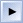# The numbers from 1 to 99.Add, substract, multiply and divide

## Click onto hear an arithmetic operation (again). Then click on the corresponding result.

### Haz clic enpara escuchar (otra vez) un cálculo.Después haz clic en el resultado que se corresponde con el cálculo.Haz clic en Next calculation para escuchar otro cálculo.

 How to calculate in English 2 + 5 (= 7) → two plus five (equals seven) 7 - 2 (= 5) → seven minus two (equals five) 2 × 4 (= 8) → two multiplied by four (equals eight) 8 ÷ 2 (= 4) → eight divided by two (equals four)
 7 8 10 15 21 23
 36 42 47 51 54 62
 66 73 77 85 93 98
You've made all the calculations of this exercise. Now we're going to present you the same calculations in a different order.